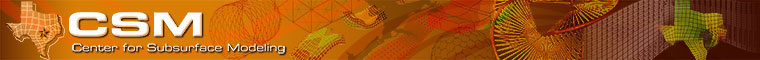Introduction Source code Documentation Build Run

 Model Keywords Set an input file keyword for your model Example: THERMAL Assign a model number to your model Example: MODEL = 19 Use the keyword and model number to register the model in IPARSv2/input/idata.df Set a model keyword for preprocessing Example: \$THERMAL All your model dependent calls from the framework routines should be hidden under this keyword \$THERMAL CALL TMSTEP( ) Make a directory for your model Example: IPARSv2/thermal/ Name your files to distinguish from other models Example: thivdat.df, thbwell.df, thstep.df Create a model makefile and size file Example: thermal.mak, thermal.siz Make a directory for your model Example: in IPARSv2/ipars.df \$BLACKP IF (MODEL.EQ.1) CALL PSTEP(NERR) \$BLACKI IF (MODEL.EQ.2) CALL ISTEP(NERR) ... \$TRCHEM IF (MODEL.EQ.17) CALL TRSTEP(NERR) \$POROE IF (MODEL.EQ.18) CALL PESTEP(NERR) \$THERMAL IF(MODEL.EQ.19) CALL THSTEP(NERR)

Written by Center for Subsurface Modeling